What does it do?

Gets the max value based on the cells that matches the criteria

Formula breakdown:

=MAXIFS(max_range, criteria_range1, criteria1, …)

What it means:

=MAXIFS(cells that contains the values, first set of cells to base the filtering on, filtering condition of first set of cells, …)

If you need to get the max value while doing filtering at the same time, the MAXIFS Formula will do this for you in Excel! This was introduced in Excel 2019.

You need to specify on which ones you want to get the MAX value, then specify one or more conditions used for filtering. In our example, we want to get the maximum sales of John!

I explain how you can do this below:STEP 1: We need to enter the MAXIFS function in a blank cell:

## =MAXIFS(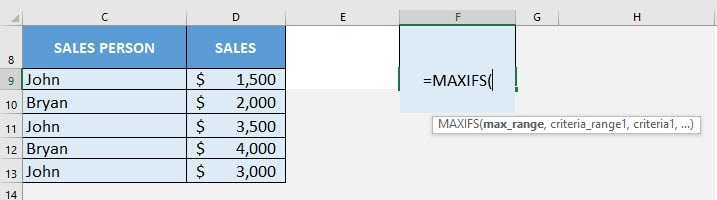STEP 2: The MAXIFS arguments:

## max_range

What is the range that contains the values to get the max value?

Select the cells containing the sales numbers that you want to get the maximum value from:

## =MAXIFS(D9:D13,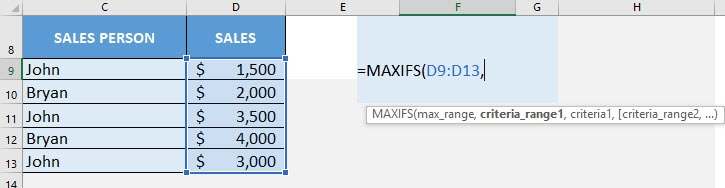## criteria_range1

What is the range that contains the values for filtering?

Select the cells containing the sales person names:

## =MAXIFS(D9:D13, C9:C13,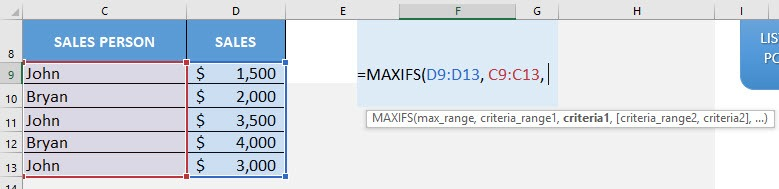## criteria1

What is the your filtering criteria?

Since we want to filter to the sales numbers of John, type in John:

## =MAXIFS(D9:D13, C9:C13, “John”)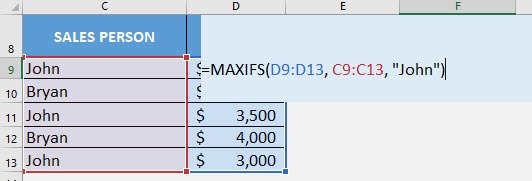You now have John’s highest sales number!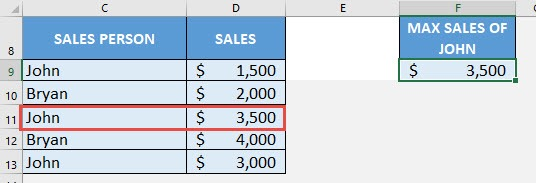How to Use the MAXIFS Formula in Excel